# Multiples of 6

Multiples of 6• The multiples of 6 are numbers that can be divided exactly by 6, leaving no remainder.
• The first few multiples of 6 are the numbers in the 6 times table.
• For example 2 × 6 = 12 and so, the second multiple of six is 12.
• For example 100 × 6 = 600 and so, the hundredth multiple of 6 is 600.
• A number is a multiple of 6 if it can be divided by both 2 and 3.
• This means that all multiples of 6 are even, ending in 0, 2, 4, 6, or 8.

A multiple of 6 is any number that can be divided by 6 exactly, leaving no remainder.#### Multiples of 6 Flashcards

Click on the multiples of 6 flashcards below to memorise the 6 times table:

×
×

=#### Multiples of 6: Interactive Questions

Multiples of 6: Interactive Questions

# Multiples of 6

## What are Multiples of 6?

Multiples of 6 are numbers that can be divided exactly by 6. Multiples of 6 are made by multiplying a whole number by 6. For example, the tenth multiple of 6 is 60 because 10 × 6 = 60.

Multiples of 6 are made by multiplying any whole number by 6. Therefore, the first multiples of 6 are the numbers in the 6 times table.The first few multiples of 6 are:

• 1 × 6 = 6
• 2 × 6 = 12
• 3 × 6 = 18
• 4 × 6 = 24
• 5 × 6 = 30
• 6 × 6 = 36
• 7 × 6 = 42
• 8 × 6 = 48
• 9 × 6 = 54
• 10 × 6 = 60
• 11 × 6 = 66
• 12 × 6 = 72

Here are the multiples of 6 shown on a number chart.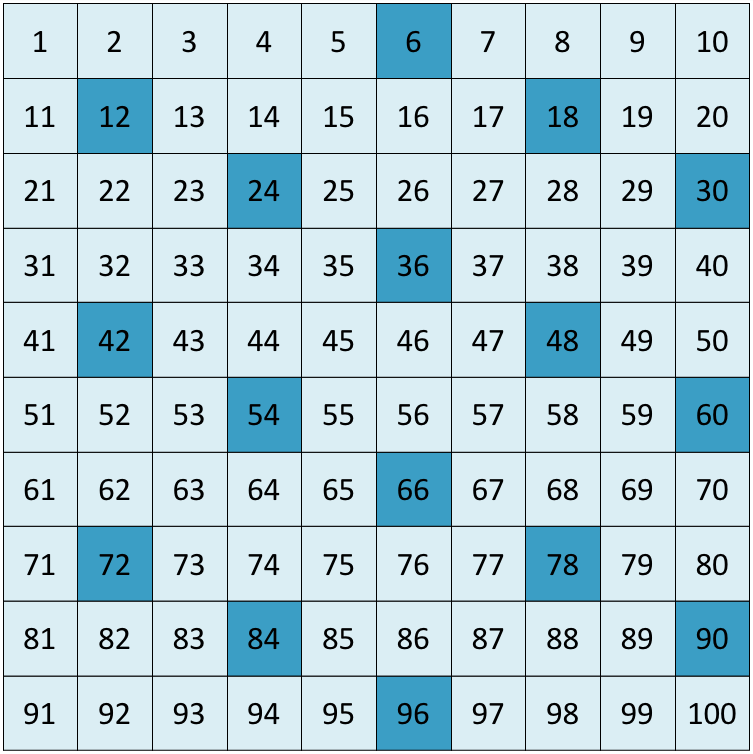6 can be divided exactly by 2 and 3. This means that all multiples of 6 are also multiples of 2 and multiples of 3.

Multiples of 6 are always even.

## What are the Multiples of 6 to 100

There are 16 multiples of 6 that are less than 100. They are:

6, 12, 18, 24, 30, 36, 42, 48, 54, 60, 66, 72, 78, 84, 90 and 96.

Here is a list of the first 100 multiples of 6:

6, 12, 18, 24, 30, 36, 42, 48, 54, 60, 66, 72, 78, 84, 90, 96, 102, 108, 114, 120, 126, 132, 138, 144, 150, 156, 162, 168, 174, 180, 186, 192, 198, 204, 210, 216, 222, 228, 234, 240, 246, 252, 258, 264, 270, 276, 282, 288, 294, 300, 306, 312, 318, 324, 330, 336, 342, 348, 354, 360, 366, 372, 378, 384, 390, 396, 402, 408, 414, 420, 426, 432, 438, 444, 450, 456, 462, 468, 474, 480, 496, 492, 498, 504, 510, 516, 522, 528, 534, 540, 546, 552, 558, 564, 570, 576, 582, 588, 594, 600.

## How to Find Multiples of 6

To find a multiple of 6, multiply a whole number by 6. For example, 3 × 6 = 18 and so, 18 is the third multiple of 6. Alternatively, start at 0 and count up in sixes. We get 6, 12, 18, 24, 30 and so on.

The first 12 multiples of 6 are shown in the poster below.To find the next multiples of 6, simply add on 6.

For example, 72 + 6= 78, 78 + 6 = 84 and 84 + 6 = 90.

Therefore 78, 84 and 90 are the thirteenth, fourteenth and fifteenth multiples of 6 respectively.

Here are the remaining multiples of 6 shown on a number grid. When teaching the multiples of 6, start at 0 and count up in sixes. A number grid can be useful for looking for patterns in the multiples and can assist with adding 6 to larger numbers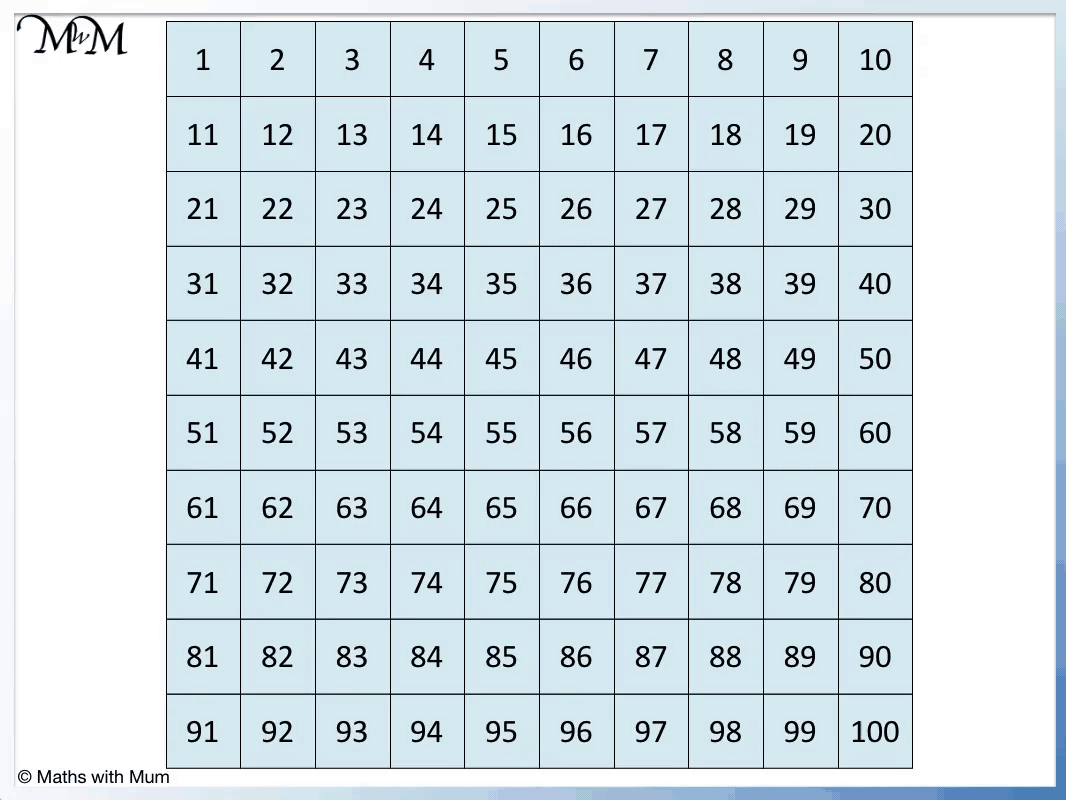We can see that multiples of 6 always end in 2, 4, 6, 8 or 0. They do not end in any other number. For a number to be a multiple of 6, it must be an even number.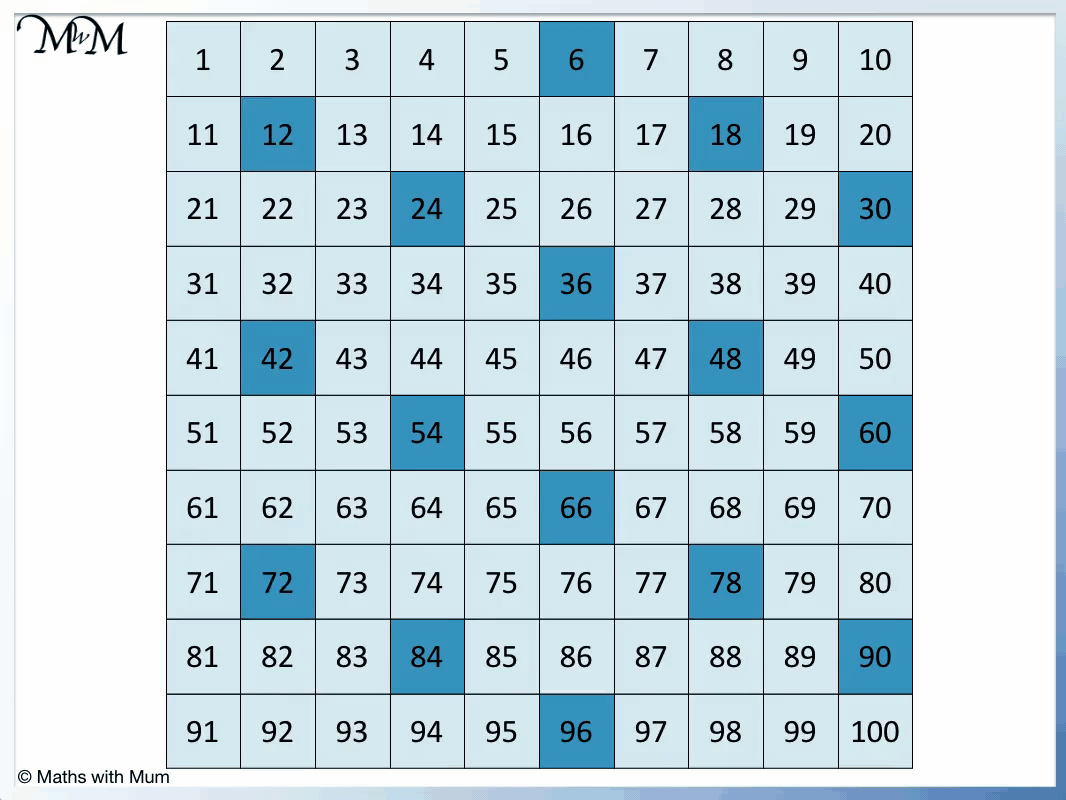## The Rule for Recognising Multiples of 6

The rule for recognising multiples of 6 is that the number must end in 0, 2, 4, 6, or 8 and the digits must add up to make a number in the 3 times table. For example, 318 is a multiple of 6 because it ends in an 8 and the digits 3 + 1 + 8 add to make 12, which is a number in the 3 times table.

Multiples of 6 are also multiples of 2 and multiples of 3. This is because 6 can be divided exactly by 2 and by 3.

Therefore to test if a number is a multiple of 6 or not, it must also be a multiple of 2 and a multiple of 3.

Multiples of 2 are even numbers and must end in a 0, 2, 4, 6 or 8.

All multiples of 3 have digits that add up to multiples of 3.

A number must pass both of these tests if it is a multiple of 6.

For example, here is 318.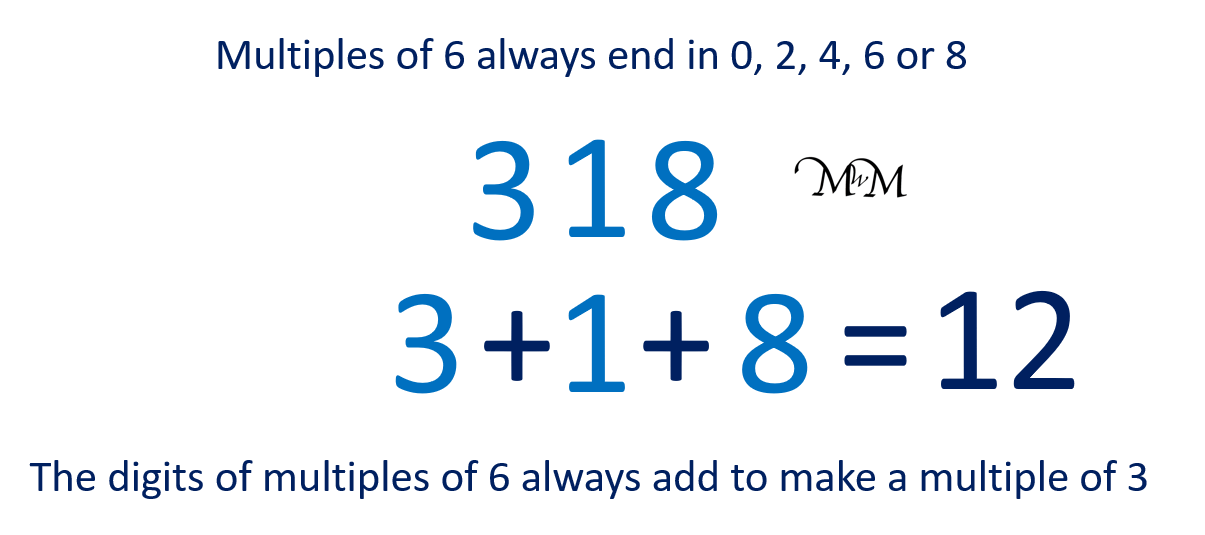It is a multiple of 2 because it ends in an 8. Multiples of 2 end in 0, 2, 4, 6 or 8.

It is a multiple of 3 because its digits add up to 12. 12 is in the 3 times table.

318 is a multiple of 2 and 3 and therefore, it is a multiple of 6.

If the number does not pass both of these tests, it is not a multiple of 6.

Here is another example of 564.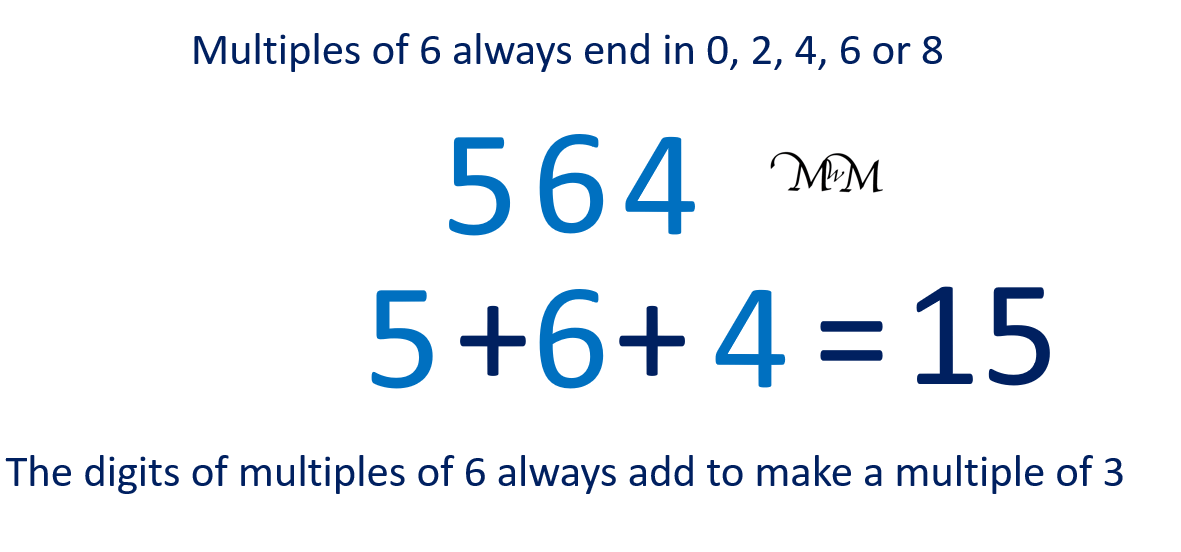564 ends in a 4. 4 is an even number and so, 564 is a multiple of 2.

5 + 6 + 4 = 15, which is in the 3 times table. 564 is a multiple of 3.

564 is a multiple of 2 and a multiple of 3, therefore it is also a multiple of 6.Now try our lesson on Lattice Multiplication Method where we learn how to use the lattice method for multiplying numbers.error: Content is protected !!